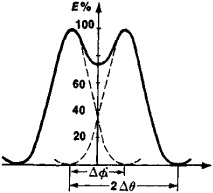# Resolving Power of Optical Instruments

The following article is from The Great Soviet Encyclopedia (1979). It might be outdated or ideologically biased.

## Resolving Power of Optical Instruments

a quantity that characterizes the ability of optical instruments to produce separate images of two points of an object that are close to each other. The smallest linear or angular distance between the two points at which their images begin to merge is called the linear or angular limit of resolution. The inverse quantity usually serves as a quantitative measure of the resolving power.

Because of the diffraction of light at the edges of optical components, even in an ideal optical system (that is, one without aberrations), the image of a point is not a point but a central disk of light surrounded by rings, which are alternately dark and light in monochromatic light and rainbow-colored in white light. The theory of diffraction makes it possible to calculate the shortest distance resolvable by a system if it is known for what intensity distributions the detector (such as the eye or a photosensitive layer) perceives the images separately. According to Lord Rayleigh (1879), the images of two points of identical brightness can still be seen separately if the center of the diffraction image of each is intersected by the edge of the first dark ring of the other (see Figure 1). In the case of point sources emitting noncoherent rays, when the above criterion (Rayleigh criterion) is satisfied, the lowest illumination between the images of resolvable points will be 74 percent of the maximum value, and the angular distance between the centers of the diffraction images (the intensity maxima) will be ΔΦ = 1.21λ/D, where λ is the wavelength of light and D is the diameter of the entrance pupil of the optical system. If f is the focal length of the optical system, then the linear value of the Rayleigh limit of resolution is σ = 1.21λf/D.

The limit of resolution of telescopes and optic tubes is expressed in seconds of arc; for the wavelength λ 560 nanometers (corresponding to the maximum sensitivity of the human eye), it is equal to α″ = 140/D where D is given in mm. For photographic objectives, the resolving power is usually defined as the maximum number of distinctly visible lines per millimeter of image of a standard test object; it is calculated by the formula N = 1,470∊, where c is the relative aperture of the objective. These relations are valid only for points located on the axisFigure 1. Intensity distribution E in the image of two point sources of light situated so that the angular distance ΔΦ between the intensity maxima is equal to the angular value Δθ of the radius of the central spot of the diffraction image (ΔΦ = Δθ is a condition imposed by Lord Rayleigh)

of an ideal optical system. The presence of aberrations and manufacturing defects increases the size of diffraction images and lowers the resolving power of real systems; the resolving power also decreases with the distance from the center of the field of view.

The resolving power of an optical instrument Ropt comprising an optical system with a resolving power Ros and an optical detector (such as a photosensitive layer or the cathode of an image converter) with a resolving power Rd is defined by the approximation formula 1/Ropt = 1/Ros + 1/Rd. It follows from this formula that it is advisable to use only combinations in which Ros and Rd are quantities of the same order.

The resolving power of an instrument can be estimated from the instrument’s spread function, which reflects all factors affecting image quality, such as diffraction and aberrations. Image quality is often estimated from the frequency-contrast curve, as well as from the resolving power.

### REFERENCES

Tudorovskii, A. I. Teoriia opticheskikh priborov, 2nd ed., part 1. Moscow-Leningrad, 1948.
Landsberg, G. S. Optika, 4th ed. Moscow, 1957. (Obshchii kurs fiziki, vol. 3.)
Volosov, D. S. Fotograficheskaia optika. Moscow, 1971.

L. N. KAPORSKII